# Basic Arithmetic : Addition with Whole Numbers

## Example Questions

### Example Question #1 : Operations With Whole Numbers

If I had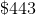in the bank and deposited an additonal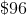today, how much do I have in total?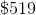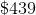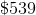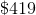Explanation: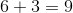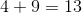***don't forget to put the 3 down and carry the one to the hundreds place***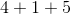### Example Question #2 : Whole Numbers

Find the sum.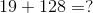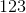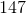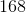Explanation:

First add the digits in the ones colume, this would be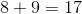. Make sure to carry the 1 from the 17 to the tens colume.

Then add the digits in the tens colume, this would be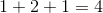.

Lastly, add the digits in the hundrends colume, this would beTherefore the result is as follows: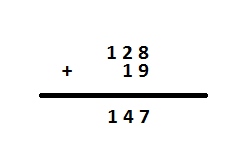### Example Question #2 : Whole Numbers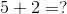What is the solution of the above operation?Explanation: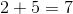We can see this because if we had 5 (represented by) and add another 2 objects (represented by) we should get 7 objects (and) total.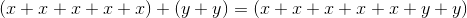We have 7 objects total!

### Example Question #3 : Whole Numbers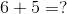What is the solution of the above equation?Explanation: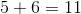If x represents objects.

For 5: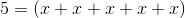For 6: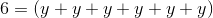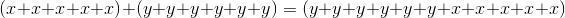We can see that 11 objects result.

### Example Question #1 : Addition With Whole Numbers

For the following questions, please select the answer that most correctly completes the equation.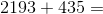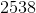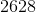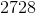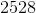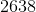Explanation: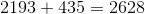Ones digit: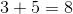Tens digit: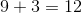(leave the two at the tens, carry the one to the hundreds digit)

Hundreds digit: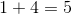, add the one from the tens digit, so you get 5 + 1 = 6

Thousands digit: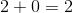### All Basic Arithmetic Resources